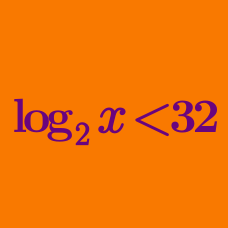Algebra

Logarithmic Inequalities: Level 3 Challenges

If the domain of the solutions of the inequality $(\log_{2}{x})^2 < 2\log_{2}{x}+3$ is from $a$ to $b$ (both exclusive). Find the value of $a+b$.

$\large \log_{0.3}{(x-1)}<\log_{0.09}{(x-1)}$

Find the range of $x$ that satisfies the inequality above.

Find the minimum value of the positive integer $x$ satisfying the inequality $\frac{\log_{10} (2x-1)}{2}+\log_{10} \sqrt{x-9} \geq 1.$

Source: RMO

Find the smallest positive integer $k$ such that $\dfrac{1}{\log_{3^k}2015!}+\dfrac{1}{\log_{4^k}2015!}+\ldots+\dfrac{1}{\log_{2015^k}2015!}>2015$

$\large \log_{x}(2x)+\log_{2}(x)\geq 3$ The inequality above has a solution in the form $(a,+\infty)$. Find the value of $a$

×